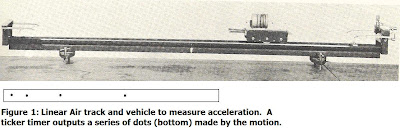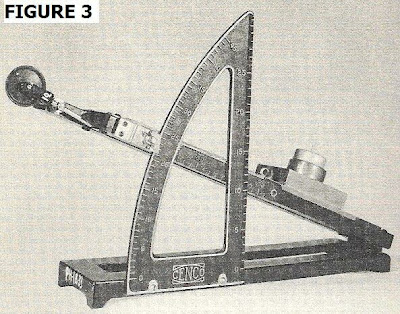## Tuesday, May 3, 2011

### Introduction to Basic Physics (Mechanics) Pt. 21. Demonstrations of the Second Law:

The simplest way to demonstrate Newton's law (2) is by using a linear air track to which a ticker tape is attached (See Fig. 1). By generating a constant acceleration, one can then obtain the numerical value by analysis of the dot spacing on the tape. Of course, several trial runs need to be performed first in order to normalize the process. In the diagram below, the linear track is shown at a slight incline as well as the tape dots produced from the motion.

From this the acceleration, a, can be computed knowing the tape imprints a dot every 1/50 sec or 0.02s (such as we used in Barbados based on a 50 Hz supply). From this we can ascertain a uniform acceleration a = 3 m/s/s. Other demonstrations can be made by modifying the demonstrations of Figs. 1, 2 of the previous Mechanics instalment. For example, if we exert an applied force F that is adequate to overcome the frictional force f, then we obtain a net resultant force (F – f) > 0, and so Newton’s 2nd law will now apply. Similarly, in the demonstration for Fig. 2 (previous blog instalment), if we now attach a slight mass m’ to m2 so that now: (m2 + m’) > m1 we will find a net motion in the direction of m2, and hence a net resultant force such that:

(m2 + m’)g > m1 g so that: T2 > T1

Similarly, one can make inferences on how the graphs of motions will change, say if the tape data from the linear air track experiment is used as opposed to the uniform motion from Fig. 3. If then, we have an acceleration: a = 3 m/s then v = at = 3t and clearly the slope (v2 – v1)/ delta t is no longer constant. Thus:

m (v2 – v1)/ delta t = ma = F

confirming the 2nd law.

The key here is that we’re now dealing with an unbalanced force.

2. Deducing whether the 1st or 2nd law applies from analysis of the motion.

One of the most general applications of mechanics is for analysis motions of bodies and determining whether they are in uniform or accelerated motion. Graphing of the motions can easily enable us to determine those cases for which the 1st law (uniform motion) applies, as shown in Fig. 2. The top graph gives the displacement s in terms of the time as: s = 2t. The bottom graph gives the velocity based on this motion, which will be: v = s/t = 2t/t = 2, say in meters per second. Since there is no initial velocity, u = 0, then (v – u)/ t = 0 and the slope the v-t graph is zero. Meanwhile, the graph of the s-t graph (top) has constant slope. In either of these instances one can infer there is uniform motion and hence Newton’s 1st law of motion applies.

3. Another experimental setup to analyze acceleration (the inclined plane).

Another experimental demonstration which can be done uses an adjustable inclined plane for which the angle of incline,Θ , can be set. This device is shown In Fig. 3 with a mass (‘car’) on it.

The benefit of this device is that it not only can be used to demonstrate the 2nd law, but also the transition conditions from the 1st to the 2nd laws. For example, start the apparatus such that the car is in equilibrium and no motion is evident. Then it means that THREE forces are in balance in a condition of impending motion which will be in accord with the 2nd law. The diagram of this equilibrium, for which the 1st law applies, is shown in Fig. 4.

The trick then is to find the force F to just nudge the mass into sliding. This will be the force of static friction. Before this slide occurs, the three main forces are in balance as shown in the portion of diagram to the right – hence Newton’s 1st law applies. From this the coefficient of static friction can be found such that: u_s = F /N = tan Θ , where N denotes the normal force. Once the mass begins to slide then we have the resultant force acting down the incline and along it:

F = mg sin Θ = ma

Problems:

1)A car moves at 10 m/s for a period of 5 seconds then slows to 5 m/s over the next 10 seconds. Explain the changes in motion and which, if any, of Newton’s laws apply during the stages of the motion, giving reasons.

2)For the demonstration of Newton’s 1st law in Fig.1 (previous instalment), let f = u_k N, where N is the normal force and u_k is the coefficient of kinetic friction. If the weight of the mass being moved is 1 kg and u_k = 0.1, what is the smallest magnitude of the applied force F needed to still allow the 1st law to apply?